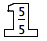### Home > CC1MN > Chapter 7 Unit 7 > Lesson CC1: 7.3.2 > Problem7-100

7-100.

For each of the following pairs of fractions, complete the fraction on the right so that the two fractions are equivalent. Using a Giant One might be helpful.

1. $\frac { 30 } { 35 } = \frac { 6 } { \square }$

The Giant One for this problem could look like this:Since $35\div5=7$, therefore:

$\frac{30}{35}= \frac{6}{7}$

1. $\frac { 8 } { 40 } = \frac { \square } { 5 }$

Make a Giant One like in part

1. $\frac { 15 } { 20 } = \frac { \square } { 4 }$

The Giant One for this problem could look like this: